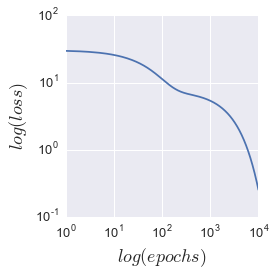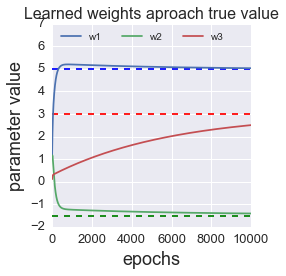# Gradient descent by matrix multiplication

Posted on Thu 23 February 2017 in blog

Deep learning is getting so popular that even Mark Cuban is urging folks to learn it to avoid becoming a "dinosaur". Okay Mark, message heard, I'm addressing this guilt trip now. I originally tried starting in tensorflow (tensors are multidimensional arrays), but I quickly realized that I don't think in terms of tensors/matrices. For example, I drew a blank when thinking about how to take a partial derivative using matrix multiplication. So, as an exercise to understand concepts such as notation and matrix computations, my goal is to implement gradient descent on a multiple regression model.

Gradient descent is fairly intuitive. The goal of gradient descent is to find the minimum of the objective function, in this case, the sum-of-squares error. A derivative tells you the slope of a function. So, just walk down (descent) the slope and you will eventually reach the bottom. The "gradient" in gradient descent is a technical term, which refers to the partial derivative of the objective function across all the descriptors. If this is new, check out the excellent descriptions by Andrew Ng and or Sebastian Rashka, or this python code. While these were helpful in gaining intuition, tensorflow uses array multiplication, and not temporary variable assignment and for loops across variables.

So let's get started.

In :
%matplotlib inline
import numpy as np
import pandas as pd

import matplotlib.pylab as plt
import seaborn as sns
import matplotlib as mpl
label_size = 13
mpl.rcParams['xtick.labelsize'] = label_size
mpl.rcParams['ytick.labelsize'] = label_size
mpl.rcParams.update({'figure.autolayout': True})


## notation and dimensionality¶

If you are familiar with matrix notation, feel free to skip this section. Otherwise, to orient you, The model we are building is a multi-dimensional linear model with the following form:

$$y=XW + bias + epsilon$$

where $X$ (n x p) is the observed data, W $(p \times 1)$ is the weights, $y$ $(n \times 1)$ is the predicted value and epsilon $(n \times 1)$ is the error. This is called the design matrix. Each data point is represented by a $(p \times 1)$ vector, so to add new data to the matrix, transpose the p-vector and append it to the bottom of the design matrix. After matrix multiplication, $XW$ is $n \times 1$, and to generate realistic data, I add an error term, epsilon. This gives enough for us to create our target data, $y$.

### generate target data $y$¶

In :
X_grid = np.mgrid[0:10:1, 0:10:1].reshape(2,-1).T # from http://stackoverflow.com/a/32208788/4691861
X = np.concatenate((X_grid,np.tile(1,[100,1])),axis=1)
true_weights = np.array([,[-1.5],])
epsilon = np.random.normal(loc=0,scale=1,size=[100,1])
y = np.matmul(X,true_weights)+epsilon


### combine the bias into $X$¶

Now the goal of gradient descent is to iteratively learn the true weights. A quick note is that the bias term can be conveniently wrapped into $XW$ by right-appending a column of ones to X and appending another predictor to the bottom of W. This makes sense if you consider $(n \times p+1) \times (p \times 1) = n \times 1$. The reason it works becomes clear after doing the derivatives.

Now, let's initialize our learned weights randomly

In :
learned_weights = np.random.normal(loc=1,scale=1,size=[3,1])
orig_learned_weights = learned_weights.copy() # copy for reference


### define the gradient¶

Our first step is to define the gradient of the objective function. The objective function we optimize is the sum-of-squares error between the true and learned weights. $$obj={\dfrac{1}{2}} \sum\limits_{i=i}^{n} (\hat{y}-y)^2$$ which can be rewritten as $$obj={\dfrac{1}{2}} \sum\limits_{i=i}^{n} (XW-y)^2$$ Then $$\nabla obj=\sum\limits_{i=i}^{n} (XW-y)D(XW)$$ where $D$ refers to the matrix derivative, in our case, with respect to W.

So at each epoch (training iteration), we will update each weight according to

$$w_j=w_j-learning\:rate \times \nabla obj_j$$

Thus at each step, we update each weight by moving opposite the gradient (subtraction) toward the minimum of the objective function by a step size defined by the learning rate.

### variable updates through matrix multiplication¶

How do we do the variable updates using matrices? Well, first we need to understand how matrix derivatives work.

I found that writing out an example was helpful. Consider two arbitrary matrices $X_{3 \times 2}$ and $W_{2 \times 1}$ of the form

$$X=\begin{bmatrix} x_1 & x_4\\ x_2 & x_5\\ x_3 & x_6\\ \end{bmatrix}$$$$W=\begin{bmatrix} w_1\\ w_2\\ \end{bmatrix}$$

Then

$$XW=\begin{bmatrix} x_1w_1 + x_4w_2\\ x_2w_1 + x_5w_2\\ x_3w_1 + x_6w_2\\ \end{bmatrix}$$

Taking the partial derivative of each weight gives

$$\frac{\partial{XW}}{\partial w_1}=\begin{bmatrix} \frac{\partial{(x_1w_1 + w_4w_2)}}{\partial w_1}\\ \frac{\partial{(x_2w_1 + x_5w_2)}}{\partial w_1}\\ \frac{\partial{(x_3w_1 + w_6w_2)}}{\partial w_1}\\ \end{bmatrix} =\begin{bmatrix} x_1\\ x_2\\ x_3\\ \end{bmatrix}$$$$\frac{\partial{XW}}{\partial w_2}=\begin{bmatrix} \frac{\partial{(x_1w_1 + x_4w_2)}}{\partial w_2}\\ \frac{\partial{(x_2w_1 + x_5w_2)}}{\partial w_2}\\ \frac{\partial{(x_3w_1 + x_6w_2)}}{\partial w_2}\\ \end{bmatrix} =\begin{bmatrix} x_4\\ x_5\\ x_6\\ \end{bmatrix}$$

This is equivalent to choosing the j th column of $X$ for each weight. Then this can be represented in matrix form as $\frac{\partial{XW}}{\partial w_1} = X \begin{bmatrix} 1\\ 0\\ \end{bmatrix}$ and $\frac{\partial{XW}}{\partial w_2} = X \begin{bmatrix} 0\\ 1\\ \end{bmatrix}$

So we can effectively compute the parital derivatives of all weights by using a $(p+1 \times p+1)$ diagonal matrix of ones. First, let's use this trick to figure out how to compute $D(WX)$ in matrix form.

In :
ones = np.zeros((3, 3))
np.fill_diagonal(ones, 1)
diag_ones = ones.reshape((3,3,1))


Show the shape of the diag_ones matrix

In :
diag_ones

Out:
array([[[ 1.],
[ 0.],
[ 0.]],

[[ 0.],
[ 1.],
[ 0.]],

[[ 0.],
[ 0.],
[ 1.]]])

Now multiplying it through gives us

In :
deltaXW_deltaW = np.matmul(X,diag_ones)


Then, check that the dimensions match...

In :
print deltaXW_deltaW.reshape((3,100)).shape
y_hat_minus_y = np.matmul(X,learned_weights) - y
print y_hat_minus_y.shape

(3, 100)
(100, 1)


Voila! The matrices are of the right dimension to compute the gradients across all weights simultaneously. Now we can perform $$w_j=w_j-learning\:rate \times \nabla obj_j$$ using matrix multiplation

### Ready for liftoff¶

Now, we are ready to watch the learning happen. First, we will define a learning rate, initiate weights randomly, perform the updates, and compare them to the defined weights we picked arbitrarily.

In :
learning_rate = 0.001
num_samples = X.shape
learned_weights=orig_learned_weights.copy()


### perform gradient descent over 10,000 epochs¶

We will track the $loss$ (the squared error) and $W_{learned}$ after each iteration, then see how the algorithm performed

In :
epoch_num = 10000
weight_updates = np.zeros((epoch_num + 1,3))
loss = np.zeros(epoch_num + 1)
loss = np.sum(np.square(true_weights - learned_weights))
for epoch in range(epoch_num):
y_hat_minus_y = np.matmul(X,learned_weights) - y
learned_weights = learned_weights - gradient_step
loss[epoch+1] = np.sum(np.square(true_weights - learned_weights))

In :
fig, ax = plt.subplots(figsize=(4,4))
ax.loglog(loss)
ax.set_xlabel('$log(epochs)$',fontsize=18)
ax.set_ylabel('$log(loss)$',fontsize=18)
plt.show()As shown in the plot above, the loss is monotonically decreasing over all epochs. Thus, the algorithm effectively descends the gradient to the true weights. Let's see how that happens by plotting each of the three weights

In :
fig, ax = plt.subplots(figsize=(4,4))
ax.hlines(5,0,10000,linestyles='dashed',color='blue')
ax.hlines(3,0,10000,linestyles='dashed',color='red')
ax.hlines(-1.5,0,10000,linestyles='dashed',color='green')
ax.set_ylim([-2,7])
ax.set_xlabel('epochs',fontsize=18)
ax.set_ylabel('parameter value',fontsize=18)
ax.legend(('w1','w2','w3'),loc=2,ncol=3)
ax.set_title('Learned weights aproach true value',fontsize=16)
plt.show()Each weight asymptotically approaches the true value (indicated by dashed line), validating the approach. As an aside, increasing the learning rate by a factor of 50 ($learning\:rate=0.05$) gives an overflow error, so some thought should be given as to what it should be set to

### How much faster is matrix implementation than for loops?¶

So, how much was the exercise in math worth it? How much faster is the algorithm than using temporary variable assignment and nested for loops?

##### Matrix implementation¶
In :
%%time
for epoch in range(int(1e5)):
y_hat_minus_y = np.matmul(X,learned_weights) - y
learned_weights = learned_weights - gradient_step
#print np.sum(np.square(true_weights - orig_learned_weights))
#print np.sum(np.square(true_weights - learned_weights))

CPU times: user 733 ms, sys: 2.44 ms, total: 735 ms
Wall time: 734 ms

##### for loop implementation¶
In :
%%time
# Initialize variables
learned_weights = orig_learned_weights.copy()
y_hat_minus_y = np.zeros((num_samples,1))

CPU times: user 6.5 s, sys: 8.09 ms, total: 6.51 s